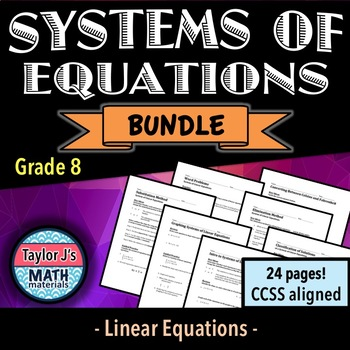# Systems of Equations Worksheet Bundle7th - 10th
Subjects
Standards
Resource Type
Formats Included
• Zip
•Activity
Pages
48 pages
\$12.15
Bundle
List Price:
\$13.50
You Save:
\$1.35
\$12.15
Bundle
List Price:
\$13.50
You Save:
\$1.35
Easel Activities Included
Some resources in this bundle include ready-to-use interactive activities that students can complete on any device. Easel by TpT is free to use! Learn more.

### Description

Students solve systems of equations by graphing, substitution method and elimination method; word problems included.

**If you only need one of the lessons, each is sold individually!

What is covered:

- It reviews the following vocabulary and key terms: system of linear equations, slope-intercept form, solution to a system of equations, classification of solutions (one, infinite or no solutions), substitution method, elimination method

Worksheet Breakdown:

1. Introduction to Systems of Equations Worksheet: Students find the solution to a system of linear equations by graphing; word problems included

2. Graphing Systems of Equations Worksheet: Students find the solution to a system of linear equations by graphing

3. Classification of Solutions of Systems of Equations Worksheet: Students determine whether a system has one solution, infinite solutions, or no solutions

4. Substitution Method Worksheet: Students use the substitution method to solve a system of equations algebraically

5. Elimination Method Worksheet: Students use the elimination method to solve a system of equations algebraically

6. Word Problems Worksheet: Students use the elimination and substitution method to solve word problems involving a system of equations

7. Converting Between Celsius and Fahrenheit Worksheet: Students use a formula to convert between celsius and fahrenheit

Possible uses:

- End-of-topic cumulative review guide

- Extra practice for struggling students

- Homework

- Test prep

- Bellringer or warm ups

*************************************************************************************************************

You might be interested in:

- these FREEBIES

- the unit on Congruence

- the unit on Linear Equations

- the unit on Percents & Proportional Relationships

or my store for other material to supplement the 7th & 8th grade curriculum!

Follow My Store to receive email updates on new items, product launches, and sales!

**If you have any requests and/or updates for my work, please message me!

Total Pages
48 pages
Included
Teaching Duration
N/A
Report this resource to TpT
Reported resources will be reviewed by our team. Report this resource to let us know if this resource violates TpT’s content guidelines.

### Standards

to see state-specific standards (only available in the US).
Graph proportional relationships, interpreting the unit rate as the slope of the graph. Compare two different proportional relationships represented in different ways. For example, compare a distance-time graph to a distance-time equation to determine which of two moving objects has greater speed.
Use similar triangles to explain why the slope m is the same between any two distinct points on a non-vertical line in the coordinate plane; derive the equation 𝘺 = 𝘮𝘹 for a line through the origin and the equation 𝘺 = 𝘮𝘹 + 𝘣 for a line intercepting the vertical axis at 𝘣.
Analyze and solve pairs of simultaneous linear equations.
Understand that solutions to a system of two linear equations in two variables correspond to points of intersection of their graphs, because points of intersection satisfy both equations simultaneously.
Solve systems of two linear equations in two variables algebraically, and estimate solutions by graphing the equations. Solve simple cases by inspection. For example, 3𝘹 + 2𝘺 = 5 and 3𝘹 + 2𝘺 = 6 have no solution because 3𝘹 + 2𝘺 cannot simultaneously be 5 and 6.

### Questions & Answers

Teachers Pay Teachers is an online marketplace where teachers buy and sell original educational materials.